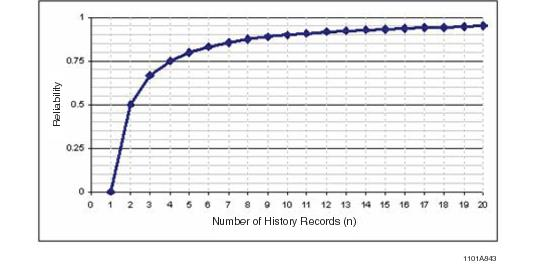# 15.10 - Determining the Reliability of Statistics From History Intervals - Teradata Database

## Teradata Database SQL Request and Transaction Processing

prodname
vrm_release
15.10
category
Programming Reference
User Guide
featnum
B035-1142-151K

Teradata Database performs linear trend analyses (linear regression) of historical demographics to determine the reliability factor for downgrading to sampled statistics, applying threshold techniques, stale statistics extrapolations. The reliability factor is derived from a combination of various stability and consistency factors.

Teradata Database retains history records and analyzes changes in the trends of the cardinalities for collected statistics. The Optimizer attempts to find a linear relationship between each statistic and its cardinality trends. If it finds a reliable linear relationship, it models the relationship as a linear function. Teradata Database uses the linear functions it develops to estimate the cardinality for a current statistic at a given time. If the Optimizer does not find a reliable trend, it uses heuristics to estimate the cardinality of a statistic. When the historical change trends for statistics do not match their recent changes, Teradata Database purges the past statistics automatically.

This approach assumes that the current and future changes of statistics will be similar to any recent changes. For situations when that assumption is clearly not valid, such as when newly added or updated data is significantly different from recent past insertions deletions, or updates, you should recollect statistics.

The history of a statistic is a set of history records. Teradata Database maintains 2 types of statistics history records.

• Those based on timestamps and cardinalities
• Those based on statistics values and cardinalities
• Among the historical statistics values Teradata Database maintains are the number of unique values, the number of nulls, the high modal frequency, the minimum value, and the maximum value.

The criteria Teradata Database uses to select a single best trend line from the available candidate trend lines are as follows.

• The trend line must be derived from the most recent history records
• The trend line must be stable
• The trend line must be consistent
• The reliability of an estimated statistical value is determined by a weighting function. A reliability weighting function with values that range between 0 and 1 represents how reliable a statistic computed using a linear regression method is.

Teradata Database computes this reliability based on information it derives from stability and consistency estimates made for the most recent trend. Given a stability threshold, a trend analysis locates all of the recent trends that satisfy a given stability threshold.

Based on a rule that states that a trend line is stable if its stability value between the bounds of 0 and 1 is greater than some specified threshold, Teradata Database selects the trend that has the largest number of history records.

The following graph shows a trend analysis where the trend line indicates that there should be a minimum of 10 history intervals that satisfy the stability threshold to reach a reliability value greater than 0.9.When history data is available, Teradata Database extrapolates or interpolates statistics values using linear regression.

For example, Teradata Database uses linear regression to estimate various measures of a value and then combines them to estimate a statistical value.